## Saturday, November 20, 2010

### Introduction:

Don't be confused with the title, this article has nothing to do with "how to calculate an exponent of a given matrix", rather it will discuss on how to use this technique to solve a specific class of problems.

Sometimes we face some problems, where, we can easily derive a recursive relation (mostly suitable for dynamic programming approach), but the given constraints make us about to cry, there comes the matrix exponentiation idea. The situation can be made more clear with the following example:

Let, a problem says: find f(n) : n'th Fibonacci number. When n is sufficiently small, we can solve the problem with a simple recursion, f(n) = f(n-1) + f(n-2), or, we can follow dynamic programming approach to avoid the calculation of same function over and over again. But, what will you do if the problem says, given 0 < n < 1000000000, find f(n) % 999983 ? No doubt dynamic programming will fail!

We'll develop the idea on how and why these types of problems could be solved by matrix exponentiation later, first lets see how matrix exponentiation can help is to represent recurrence relations.

### Prerequisite:

Before continuing, you need to know:

• Given two matrices, how to find their product, or given the product matrix of two matrices, and one of them, how to find the other matrix.
• Given a matrix of size d x d, how to find its n'th power in O( d3log(n) ).

### Patterns:

What we need first, is a recursive relation, and what we want to do, is to find a matrix M which can lead us to the desired state from a set of already known states. Let, we know k states of a given recurrence relation, and want to find the (k+1)th state. Let M be a k x k matrix, and we build a matrix A:[k x 1] matrix from the known states of the recurrence relation, now we want to get a matrix B:[k x 1] which will represent the set of next states, i.e. M x A = B, as shown below:

 ` | f(n) | | f(n+1) | | f(n-1) | | f(n) |M x | f(n-2) | = | f(n-1) | | ...... | | ...... | | f(n-k) | |f(n-k+1)|`

So, if we can design M accordingly, job's done!, the matrix will then be used to represent the recurrence relation.

• #### Type 1:

Lets start by the simplest one, f(n) = f(n-1) + f(n-2).
So, f(n+1) = f(n) + f(n-1)
Let, we know, f(n) and f(n-1); we want to get f(n+1)
From the above situation, matrix A and B can be formed as shown below:

 Matrix A Matrix B `| f(n) || f(n-1) |` `| f(n+1) || f(n) |`

[Note: matrix A will be always designed in such a way that, every state on which f(n+1) depends, will be present]
So, now, we need to design a 2x2 matrix M such that, it satisfies M x A = B as stated above.
The first element of B is f(n+1) which is actually f(n) + f(n-1). To get this, from matrix A, we need, 1 f(n) and 1 f(n-1). So, the 1st row of M will be [1 1].
 `| 1 1 | x | f(n) | = | f(n+1) || ----- | | f(n-1) | | ------ |`

Similarly, 2nd item of B is f(n) which we can get by simply taking 1 f(n) from A. So, the 2nd row of M is [1 0].
 `| ----- | x | f(n) | = | ------ || 1 0 | | f(n-1) | | f(n) |`

[I hope you know how a matrix multiplication is done and how the values ar assigned!]
Thus we get the desired 2 x 2 matrix M:
 `| 1 1 | x | f(n) | = | f(n+1) || 1 0 | | f(n-1) | | f(n) |`

If you are confused about how the above matrix is calculated, you might try doing it this way:

We know, the multiplication of an n x n matrix M with an n x 1 matrix A will generate an n x 1 matrix B, i.e. M x A = B. The k'th element in the product matrix B is the product of k'th row of the n x n matrix M with the n x 1 matrix A in the left side.
In the above example, the 1st element in B is f(n+1) = f(n) + f(n-1). So, it's the product of 1st row of matrix M and matrix B. Let, the first row of M is [x y]. So, according to matrix multiplication,

` x * f(n) + y * f(n-1) = f(n+1) = f(n) + f(n-1)⇒ x = 1, y = 1`

Thus we can find the first row of matrix M is [1 1]. Similarly, let, the 2nd row of matrix M is [x y], and according to matrix multiplication:
` x * f(n) + y * f(n-1) = f(n)⇒ x = 1, y = 0`

Thus we get the second row of M is [1 0].

• #### Type 2:

Now, we make it a bit complex: find f(n) = a * f(n-1) + b * f(n-2), where a, b are some constants.
This tells us, f(n+1) = a * f(n) + b * f(n-1).
By this far, this should be clear that the dimension of the matrices will be equal to the number of dependencies, i.e. in this particular example, again 2. So, for A and B, we can build two matrices of size 2 x 1:

 Matrix A Matrix B `| f(n) || f(n-1) |` `| f(n+1) || f(n) |`

Now for f(n+1) = a * f(n) + b * f(n-1), we need [a b] in the first row of objective matrix M instead of [1 1] from the previous example. Because, now we need a of f(n)'s and b of f(n-1)'s.
 `| a b | x | f(n) | = | f(n+1) || ----- | | f(n-1) | | ------ |`

And, for the 2nd item in B i.e. f(n), we already have that in matrix A, so we just take that, which leads, the 2nd row of the matrix M will be [1 0] as the previous one.
 `| ----- | x | f(n) | = | ------ || 1 0 | | f(n-1) | | f(n) |`

So, this time we get:
 `| a b | x | f(n) | = | f(n+1) || 1 0 | | f(n-1) | | f(n) |`

Pretty simple as the previous one...

• #### Type 3:

We've already grown much older, now lets face a bit complex relation: find f(n) = a * f(n-1) + c * f(n-3).
Ooops! a few minutes ago, all we saw were contiguous states, but here, the state f(n-2) is missing. Now? what to do?

Actually, this is not a problem anymore, we can convert the relation as follows: f(n) = a * f(n-1) + 0 * f(n-2) + c * f(n-3), deducing f(n+1) = a * f(n) + 0 * f(n-1) + c * f(n-2). Now, we see that, this is actually a form described in Type 2. So, here, the objective matrix M will be 3 x 3, and the elements are:

 `| a 0 c | | f(n) | | f(n+1) || 1 0 0 | x | f(n-1) | = | f(n) || 0 1 0 | | f(n-2) | | f(n-1) |`

These are calculated in the same way as Type 2. [Note, if you find it difficult, try on pen and paper!]

• #### Type 4:

Life is getting complex as hell, and Mr. problem now asks you to find f(n) = f(n-1) + f(n-2) + c where c is any constant.

Now, this is a new one and all we have seen in past, after the multiplication, each state in A transforms to its next state in B.
f(n) = f(n-1) + f(n-2) + c
f(n+1) = f(n) + f(n-1) + c
f(n+2) = f(n+1) + f(n) + c
................................. so on
So, normally we can't get it through the previous fashions. But, how about now we add c as a state?

 ` | f(n) | | f(n+1) |M x | f(n-1) | = | f(n) | | c | | c |`

Now, its not much hard to design M according to the previous fashion. Here it is done, but don't forget to verify on yours:
 `| 1 1 1 | | f(n) | | f(n+1) || 1 0 0 | x | f(n-1) | = | f(n) || 0 0 1 | | c | | c |`

• #### Type 5:

Lets put it altogether: find matrix suitable for f(n) = a * f(n-1) + c * f(n-3) + d * f(n-4) + e.
I would leave it as an exercise to reader. The final matrix is given here, check if it matches with your solution. Also find matrix A and B.

 `| a 0 c d 1 || 1 0 0 0 0 || 0 1 0 0 0 || 0 0 1 0 0 || 0 0 0 0 1 |`

[Note: you may take a look back to Type 3 and 4]

• #### Type 6:

Sometimes, a recurrence is given like this:

`f(n) = if n is odd, f(n-1) else, f(n-2)In short:f(n) = (n&1) * f(n-1) + (!(n&1)) * f(n-2)`

Here, we can just split the functions in the basis of odd even and keep 2 different matrix for both of them and calculate separately. Actually, there might appear many different patterns, but these are the basic patterns.

• #### Type 7:

Sometimes we may need to maintain more than one recurrence, where they are interrelated. For example, let a recurrence relation be:
g(n) = 2g(n-1) + 2g(n-2) + f(n), where, f(n) = 2f(n-1) + 2f(n-2). Here, recurrence g(n) is dependent upon f(n) and the can be calculated in the same matrix but of increased dimensions. Lets design the matrices A, B then we'll try to find matrix M.

 Matrix A Matrix B `| g(n) || g(n-1) || f(n+1) || f(n) |` `| g(n+1) || g(n) || f(n+2) || f(n+1) |`

Here, g(n+1) = 2g(n) + 2g(n-1) + f(n+1) and f(n+2) = 2f(n+1) + 2f(n).
Now, using the above process, we can generate the objective matrix M as follows:
 `| 2 2 1 0 || 1 0 0 0 || 0 0 2 2 || 0 0 1 0 |`

So, these are the basic categories of recurrence relations which are used to be solved by this simple technique.

### Analysis:

Now that we have seen how matrix multiplication can be used to maintain recurrence relations, we are back to out first question, how this helps us in solving recurrences on a huge range.

Recall the recurrence f(n) = f(n-1) + f(n-2).

 `M x | f(n) | = | f(n+1) | | f(n-1) | | f(n) |` ............(1)

How about we multiply M multiple times? Like this:
 `M x M x | f(n) | = | f(n+1) | | f(n-1) | | f(n) |`

Replacing from (1):
 `M x M x | f(n) | = M x | f(n+1) | = | f(n+2) | | f(n-1) | | f(n) | | f(n+1) |`

So, we finally get:
 `M^2 x | f(n) | = | f(n+2) | | f(n-1) | | f(n+1) |`

Similarly we can show:

 `M^3 x | f(n) | = | f(n+3) | | f(n-1) | | f(n+2) |` `M^4 x | f(n) | = | f(n+4) | | f(n-1) | | f(n+3) |` `.............................................................................................` `M^k x | f(n) | = | f(n+k) | | f(n-1) | |f(n+k-1)|`

Thus we can get any state f(n) by simply raising the power of objective matrix M to n-1 in O( d3log(n) ), where d is the dimension of square matrix M. So, even if n = 1000000000, still this can be calculated pretty easily as long as d3 is sufficiently small.

### Related problems:

UVa 10229 : Modular Fibonacci
UVa 10870 : Recurrences
UVa 11651 : Krypton Number System
UVa 10754 : Fantastic Sequence
UVa 11551 : Experienced Endeavour

Regards, Zobayer Hasan.

1.Thank you, Zobayer.

2.Hey Zobayer. Do have any idea to solve that:
we consider F(n) function determined by following term
if n is quadratic value then F(n)=0
otherwise f(n) = [1 / {sqrt(n)}]
So what is S = F(1)+F(2)+...+F( N^2 ).
I think there is no formula exists to solve it. But How do i solve it easier??

3.I don't think I can solve this type using matrix exponentiation, I can go only for linear ones. I don't think quadratic equations can be solved.

But, still this could be solved, like, sometimes approximation formulas can be found, or, you can also try divide and conquer / dp techniques. Might help.

4.Really nice tutorial! Helped me to solve this problem on latest Codechef -
http://www.codechef.com/COOK05/problems/SEEDS/

Keep it up!

5.Hey Zobayer!
I have a problem where the recursive relation is like this:
f(n,h,k) = f(n-1,h-1,k+1) + f(n-1,h,k-1) + f(n-1,h+1,k)+1

do you have any idea how to solve this by matrix exponentiation..?

6.Is it not possible with dp? I don't think it's possible with matrix exponentiation as all of the parameters are not shifting at a same order. I am sorry if I am wrong, actually I don't know the answer of your question :(

7.Hi, Zobayar. Can you explain type-7 matrix A and B. According to me it should be
| g(n) |
A = | g(n-1) |
| f(n) |
| f(n-1) |

B = | g(n+1) |
| g(n) |
| f(n+1) |
| f(n) |

8.@Aakash, if you want to find out g(n+1) then you will be needing:

g(n+1) = 2g(n) + 2g(n-1) + f(n+1)

So, you must have f(n+1) on the left side. Hope you get it.

9.This comment has been removed by the author.

10.nice question. look carefully, you can always do something like this, say for n = 3
1 + A + A^2 + A^3
= (1 + A) + A^2(1 + A)
so divide and conquer is possible.
I have a post related to this, which u may wish to see.
http://zobayer.blogspot.com/2009/12/power-series-evaluation.html
thanks for commenting.

11.Thanks for the informative post!
It would be more helpful, if you could point out/give links to resources about finding n'th power of matrix in O(d^3 * log(n)).

12.I think, you can find b^n in log(n) right?
a recursive algorithm might look like this:
f(b, n):
----If n == 0 return 1
----If n is odd return f(b, n-1) * b;
----else return square(f(b, n/2))

See, you are multiplying b here which is definitely O(1), however, for matrix, b will be a dxd matrix, and to multiply two dxd matrix, you need O(d^3). So the actual complexity is O(d^3 lg(n))

13.hey can you tell about uva 11651 I am not able to figure it out

14.15.I tried the HDU 2802 problem using matrix exponentiation. I got TLE, so tried storing the matrices for A, A^2, A^4,... to quicken the calculation of A^n. But still got TLE!

Can this be solved using matrix exponentiation for sure? Because there is this Chinese blog, which tells there is some cycle form - http://hi.baidu.com/xiinho/blog/item/1307e6156a9be10d4b90a741.html

16.I could not do it with matrix expo either, but I have listed it here because I have found this problem in the list of matrix expo problems somewhere, can't remember now where, many days passed. Sorry!

17.f(n) = n*f(n-1) + f(n-2)

Can this be solved using matrix exponentiation ?

18.Hi Zobayer,

Can you show how to find the result for

f(n) = a* f(n-1) + b?

Thanks :)

1.This is quite simple, I think you can try the following relation
{ { a, 1 }, { 0, 1 } } * { { f(n), b } } = { f(n+1), b }

19.Hmm...the new theme is cool ;-), nice article btw. Although I knew about matrix exponentiation before but learned a couple of new things here. Very well explained too.

1.Thank you :)

20.Can we do it for recurrence having constant as a function of n.
Like for example f(n) = f(n-1) + f(n-2) + n ?

1.I don't think so, or to be precise, I can't.

2.Yes, we can do it. Just define g(n) = n => g(n) = g(n-1) + 1, g(1) = 1.
Then, f(n+1) = f(n) + f(n-1) + g(n+1) = f(n) + f(n-1) + (n+1).
This if of type 7 as given in the article. We can solve it as:

| f(n+1) | | 1 1 1 0 | | f(n) |
| f(n) | = | 1 0 0 0 | | f(n-1) |
| g(n+2) | | 0 0 1 1 | | g(n+1) |
| 1 | | 0 0 0 1 | | 1 |

We need to find out { { f(n+1) }, { f(n) }, { g(n+2) }, { 1 } }.
We know { { f(n) }, { f(n-1) }, { g(n+1) }, { 1 } }.
The matrix is
1 1 1 0
1 0 0 0
0 0 1 1
0 0 0 1

And thank you Zobayer for this wonderful article. It helped me a lot.

3.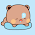21.Muhammed HedayetJune 7, 2012 at 1:28 AM

Cool. I know Matrix exponentiation technique and have solved some problems using this technique, but nice organized article. This article let me think in a new fashion. Thanks. Want more. . . :-)

1.22.Very nice post, keep up the great work.

23.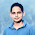I didn't know about the topic before read the article. Many many thanks for this.

24.Many many thanks to your for this article. Because the topic is new for me.... ans thanks again.

25.Many many thanks to you for this article. Because the topic is new for me........ thanks again.

26.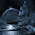good work dude..really nice

27.Thanks Vaia.It's really helpful.Thanks a lot :)

28.Thanks for sharing.
Keep up the good work !!!

29.Can we do it if we have a n on the right side?

For example:

f(n) = a*f(n-1) - n?

1.Yes we can. Just define g(n) = n => g(n) = g(n-1) + 1, g(1) = 1.
Then f(n) = a*f(n-1) - g(n). This is of type 7 as given in the article.
We need to find out the 1 column matrix:
f(n+1)
g(n+2)
1
We know:
f(n)
g(n+1)
1
The matrix is
a -1 0
0 1 1
0 0 1

2.And can we solve f(n) = a*n*f(n-1)+b*n*f(n-2)? I tried treating n like a constant but it just doesn't work. And I couldn't transform it to any type mentioned by Zobayer.

3.@Piotr Kąkol, I do not think it can be solved using matrix exponentiation methods, or at least not that I know of.

4.Yeah, I figured that if it could be, you'd make more than 7 types. Anyway, thanks for the article. Very useful!

5.Matrix exponentiation is applicable only for solving linear recurrences (and recurrences convertible to linear ones).

6.Yash you are right.

30.Superb explanation. Very clear. Thanx a ton

31.good job.....well written

32.suberb... post.. and so simple to understand.. thanx...

33.Nicely written. There aren't many good tutorials on this topic.

34.Great post!!

35.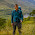Great post. I managed to solve the first 2 :)

1.Good luck with the rest too :) Thanks for visiting :)

36.Thanks a lot bro. I wanted to learn about this and at the right time found this blog.

1.Thanks for visiting, I'm glad that it helped :)

37.while(1)
printf("ধন্যবাদ,ভাইয়া :)");

38.Hi , Thanks for writing this beautiful note :)

Can you please explain, how can we build the M for the following type of function? Thanks in advance.

g(n)=g(n-1)+g(n-2)+f(n-3)
where
f(n)=f(n-1)+f(n-2)+g(n-3)

1.you can do it using a 6x6 matrix.
all you need to do is derive this matrix
f(n+1)
f(n)
f(n-1)
g(n+1)
g(n)
g(n-1)
and what you know is this matrix
f(n)
f(n-1)
f(n-2)
g(n)
g(n-1)
g(n-2)
so the matrix will be
1 1 0 0 0 1
1 0 0 0 0 0
0 1 0 0 0 0
0 0 1 1 1 0
0 0 0 1 0 0
0 0 0 0 1 0

39.I'm having trouble understanding the Formation of this matrix ...
f(n+1)
f(n)
f(n-1)
g(n+1)
g(n)
g(n-1)
I'm writing my reasoning , please correct it ......

I need to find , f(n+1) ...
f(n+1)=f(n)+f(n-1)+g(n-2)

Here , f(n+1) function's dependencies are f(n) , f(n-1) and g(n-2) ... now , I need to find out the the dependencies of g(n-2) to , get the value of g(n-2) , g(n-2)=g(n-3)+g(n-4)+f(n-5) //g() again is related to f() , I'm finding this confusing//
then , f(n+1) total dependencies are f(n),f(n-1),g(n-3),g(n-4),f(n-5) .......so , A becomes
a matrix will all the up stated values , and B will be a matrix with same functions but '1' added to the arguments . Please , Explain the fault in the reasoning a give a bit detail on what is really going on there .
Thanks .

1.Yes, there are some mistakes in your reasoning, I mean, you are correct about the dependencies, but the fact is, if you already know what g(n-2) is, you wont need to expand it further. In matrix exponentiation algorithm, with M*A = B form, the 1 column matrix you showed is B here, and A is what you already have. No need to follow recursions any more. Just try to test if recurrences hold. As I have written above, just multiply it and see for yourself you u can get the right side or not.
first row is 1 1 0 0 0 1, and the 1 column A matrix is
f(n)
f(n-1)
f(n-2)
g(n)
g(n-1)
g(n-2)
so, multiplying, we get the first row of B which is
f(n)*1 + f(n-1)*1 + f(n-2)*0 + g(n)*0 + g(n-1)*0 + g(n-2)*1
=> f(n) + f(n-1) + g(n-2)
=> f(n+1).
similarly, second row is 1 0 0 0 0 0, this is f(n)
from third row, 0 1 0 0 0 0, we get f(n-1)
from fourth row 0 0 1 1 1 0, we get f(n-2) + g(n) + g(n-1) => g(n+1)
from fifth row 0 0 0 1 0 0, we get g(n)
from sixth row, 0 0 0 0 1 0, we get g(n-1)
so, multiplying the matrix M, we get
f(n+1)
f(n)
f(n-1)
g(n+1)
g(n)
g(n-1)
Now, imagine, if we already know
f(n)
f(n-1)
f(n-2)
g(n)
g(n-1)
g(n-2)
we can then find M^n to get f(n+1) and g(n+1) at the same time, don't follow typical recurrence reasoning here. I think the later part of the post describes how this is done actually. I don't know how can I make it more clear.

2.Correction, I wanted to write this in the last:
Now, imagine, if we already know
f(2)
f(1)
f(0)
g(2)
g(1)
g(0),
then we can find M^n to get f(2+n), g(2+n) at the same time. the other values are just carried with the process.

3.Thanks , I think I got it finally .
:) I was taking the whole thing as the formal recursion and was trying to trace back and ...from f() I was trying to trace back through g() .....again f() ...all that made me confused ...

sorry but I will nag you one more time :) I'm writing another recurrence function , please say whether it's okay or not :)

Let ,
f(n)=f(n-1)+g(n-2)+h(n-3)
g(n)=g(n-1)+h(n-2)+f(n-3)
h(n)=h(n-1)+f(n-2)+g(n-3)

then ,

B will be
|f(n+1)|
|f(n) |
|f(n-1)|
|g(n+1)|
|g(n) |
|g(n-1)|
|h(n+1)|
|h(n) |
|h(n-1)|

A will be :
|f(n) |
|f(n-1)|
|f(n-2)|
|g(n) |
|g(n-1)|
|g(n-2)|
|h(n) |
|h(n-1)|
|h(n-2)|

So , M will be

| 1 0 0 0 1 0 0 0 1 |
| 1 0 0 0 0 0 0 0 0 |
| 0 1 0 0 0 0 0 0 0 |
| 0 0 1 1 0 0 0 1 0 |
| 0 0 0 1 0 0 0 0 0 |
| 0 0 0 0 1 0 0 0 0 |
| 0 1 0 0 0 1 1 0 0 |
| 0 0 0 0 0 0 1 0 0 |
| 0 0 0 0 0 0 0 1 0 |

:) Thanks

4.Yeah, its correct!

40.it realy helped me to get thrgh the concept so easily..thank u...

41.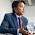Thanks Sir for this post.

42.দারুণ আইডিয়া...

43.44.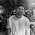That was an awesome article. However i failed to understand a certain part in type 7. I was hoping if you could explain how to arrive at this equation g(n+1) = 2g(n) + 2g(n-1) + f(n+1). from the main equation :g(n) = 2g(n-1) + 2g(n-2) + f(n), where, f(n) = 2f(n-1) + 2f(n-2).

I could form the equations for all the other cases/types ,but I don't know how to work with equations with more than one recurrence. It would be very helpful if you could explain a bit briefly.

1.Hello there, about the recursion, isn't that putting n+1 instead of n ?

45.Sorry my previous question was rather silly. I don't understand why there are 4 rows in matrix A and B. I will try to explain what i have understood. Please rectify me if I'm wrong anywhere.

Given:
g(n) = 2g(n-1) + 2g(n-2) + f(n) --->(1)
f(n) = 2f(n-1) + 2f(n-2)---->(2)

replacing n by (n+1) in equation (1).
g(n+1) = 2g(n) + 2g(n-1) + f(n+1)
RHS of the equation is the present set of states. so they should be in matrix A.
hence matrix A should be:
| g(n) |
| g(n-1) |
| f(n+1) |
and matrix B should be containing the next set of states:
| g(n+1) |
| g(n) |
| f(n+2) |

All the types from 1 to 6 were solved this way,but here in case 7 the fourth row was introduced.
Can you tell me the reason behind that or why its necessary?

1.I was hoping if you could clear my doubt mentioned above. :)

2.Sorry for late response, got carried away by the chores of daily life.
Anyway, its nothing different from the other types. Look closely, to know f(n+1), you need to know both f(n) and f(n-2). So, if you only use three rows, how are you going to get the value of f(n-2)? So, you will need two g()s and two f()s in the matrix.
Hope it is clear now. The thing is, you need to have all the dependencies on the LHS matrix.

3.Oops! sorry about the type, its not f(n-2) its f(n-1). I think you got that as well,

46.It can be done using DP, but if x and y are as large as 10^6 then one has to look around!
Matrix Expo possible?

47.It can be done using DP, but if x and y are as large as 10^6 then one has to look around!
Matrix Expo possible?

1.No, I don't think it's possible, or at least not in my knowledge.

48.Any hope with Matrix Expo? Another property of the function f(x,y) was f(x,y)=f(y,x)

1.I don't think that can be done using matrix exponentiation. If you can express one variable in terms of the other one, then some thoughts can be made, but I have no clue how to handle function on more than one variable recurrences using matrix exponentiation.

49.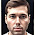Please explain Type 7 . It's given that g(n) = 2g(n-1) + 2g(n-2) + f(n) and f(n) = 2f(n-1) + 2f(n-2).
So g(n+1)=2g(n)+2g(n-1)+f(n+1) and f(n+1)=2f(n)+2f(n-1)
so shouldn't matrix A be [g(n) ,g(n-1) , f(n) , f(n-1)] .
Please explain how did you got | g(n) |
| g(n-1) |
| f(n+1) |
| f(n) |
Someone also has asked this doubt but I could not understand your explanation for that.Please explain asap.

50.Nice Article!

Can u please tell how to make approach for 11651?

Thanks

51.52.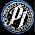zobayer why are we considering f(n)&f(n-1)in matrix exponentation after all we want to find f(n+1)

1.do you mean why we are keeping them on right hand side? they are generated as partial result, but we are not worried about them, as long as we find f(n+1).

but on the left side matrix, we need them both because f(n+1) depends on both.

53.T(n)= max ( a+ T(n-b), c+ T(n-d) ).
how can we solve this with matrix exponentiation ?

1.Seems more like dynamic programming to me. I have never tried anything of this sort, lets see if someone else can help you.

54.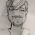Man read this topic for the first time and you nailed it. Keep it up. And thanks for writing such a brilliant post. Are there more of such useful kinds... looking forward for reading them too. Thanks

55.Hi Zobayer Hasan,
I am new to competitive programming but good at DS and Algo concepts. Can u please tell me where to start. Please share your experiences how have u mastered all tricks. How many years does it take. How much time do we need to spend coding.

56.Can you give one tutorial like this for dynamic programming. Greedy and for graph algorithms. It would be of great help

1.Well, I could find some for you searching google but then I wouldn't know if the material is good or not. To be honest, learning something deeply cannot be achieved by reading random tutorials, casually learnt knowledge does not persist much long in our brain, but the knowledge you gain when you get stuck with a problem goes a long way. My suggestion would be, do not waste time following tutorials, follow problems, and if you cant solve them, then find those solutions, you'll learn lot faster, in my opinion. :)

That being said, there are good tutorials at http://topcoder.com/tc

57.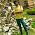Hey Can you Please explain the matrix for odd even case. I could not figure it out :(

58.hi very nice article - what about f(2n) = f(n) + f(n-1) + n for n > 1 and f(2n+1) = f(n-1) + f(n) + 1 for n >= 1 ?? any thoughts appreciated

59.oh i forgot to mention f(0) = 1 , f(1) = 1, and f(2) = 2 - thank you

60.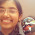hey ! Zobayer, thanks a ton man ! a

61.Just wanted to say that this was a really helpful, clearly-explained post! Great work!

62.Great Notes ,thankx.

63.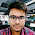It was a great Help . Thanks Man :)

64.ভাইয়া,
UVa 11651 : Krypton Number System প্রব্লেমটাতে ম্যাক্সিমাম স্কোর 1e9 না হয়ে আরো কম হলে dp দিয়ে সল্ভ করতে পারতাম, কিন্তু ওটাতে লিনিয়ার রিকারেন্স কিভাবে হচ্ছে সেটা ধরতে পারতেছি না বলে matrix exponentiation এপ্লাই করতে পারছি না... কাইন্ডলি যদি হেল্প করতেন... :)

65.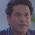f(n)=f(n-1)+f(n-2) if(n is odd)
f(n)=f(n-1)-f(n-2) if (n is even)
how can we solve this with matrix exponentiation ?

66.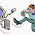ম্যাট্রিক্স এক্সপোনেনশসিয়েশন বোঝার জন্য এটা একটা সবথেকে BEST reference.. thank you!

67.Step 6 টা নিয়া যদি একটু বিস্তারিত লিখতেন তাহলে ভালো হইত (আলাদা আলাদা ব্যাপারটা বুঝিনাই) ...

68.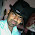http://codeforces.com/problemset/problem/821/E can you explain formation of matrix for this question